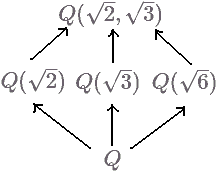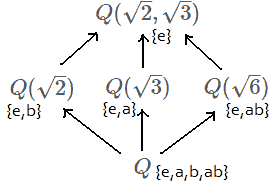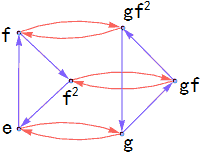# 群论系列(二)：数域的扩张

## 中间扩张## 域扩张的自同构群（伽罗瓦群）

• $K$到自身的双射（$K$中元素的一个置换操作，参看上一篇文章）；
• 保持域结构不变，结构即域上面的四则运算。比如$f(a)+f(b)=f(a+b)$，$f(a)*f(b)=f(a*b)$等；
• 额外要求（不然就和一般的域自同构群没区别了）：置换操作必须保持原域$k$不变，即对所有$a\in k$有$f(a)=a$。

• $e$：$e(\sqrt{2})=\sqrt{2}$，$e(\sqrt{3})=\sqrt{3}$（即恒映射）
• $a$：$a(\sqrt{2})=-\sqrt{2}$，$a(\sqrt{3})=\sqrt{3}$
• $b$：$b(\sqrt{3})=-\sqrt{3}$，$b(\sqrt{2})=\sqrt{2}$
• $ab$：$ab(\sqrt{2})=-\sqrt{2}$，$ab(\sqrt{3})=-\sqrt{3}$

## 子群与中间扩张

• 从下往上扩张：以最小的域$Q$为基准，考虑群$Gal(Q(\sqrt{2},\sqrt{3})/Q)$、$Gal(Q(\sqrt{2})/Q)$、$Gal(Q(\sqrt{3})/Q)$、$Gal(Q/Q)$之间的关系；
• 从上往下扩张：以最大的域$Q(\sqrt{2},\sqrt{3})$为基准，考虑群$Gal(Q(\sqrt{2},\sqrt{3})/Q(\sqrt{2},\sqrt{3}))$、$Gal(Q(\sqrt{2},\sqrt{3})/Q(\sqrt{2}))$、$Gal(Q(\sqrt{2},\sqrt{3})/Q(\sqrt{3}))$、$Gal(Q(\sqrt{2},\sqrt{3})/Q)$之间的关系。## 伽罗瓦扩张

$\theta^{3}=2$     第一个方程的解为$\theta$、$\theta\omega$、$\theta\omega^{2}$， $\omega^{3}=1$     第二个方程的解为$1$、$\omega$、$\omega^{2}$。

$$f(\theta) = \omega\theta, ; f(\omega) = \omega, \quad g(\theta) = \theta, ; g(\omega) = \omega^2.$$注意这个群不是可交换群，因为$fg = gf^2$。## 根式塔

$${\sqrt{\sqrt{2}+5}\over 1+\sqrt{\sqrt{\sqrt{3}-2}+\sqrt{7}}}$$ 这时怎样构造扩域呢？答案是：一层一层的构造。比如我们先得到了包含只允许一层根号的域$K_1$，然后我们在它的基础上再做一层根式扩张得到$K_2=K_1(\sqrt{u_1},\sqrt{u_2},..,\sqrt{u_n}), (u_1, .., u_n \in K_1)$。$K_2$就代表能用两层平方根表示的数。这样层层扩张$Q\subset K_1\subset K_2 …\subset K_n$来描述根式嵌套的方法叫根式塔

n次方程解有根式表示的意思是说，解可以用系数有限的四则运算和根式表示。我们假定系数域为k，则它等价于解落在域塔
$k\subset K_1\subset K_2 …\subset K_n$中，其中$K_{i+1} = K_i(u_i), u_i^{n}\in K_i, n\in Z$，注意我们一次只添加一个元素，如$\sqrt{2}+\sqrt{3}$认为做了两次扩张，这种看法并不影响结果，甚至我们还能要求次数n为素数（质数)，因为一个数的合数次方根可以用合数的质因数次方根的嵌套表示。

## 根式扩张的伽罗瓦群

• $u\in k$：这种情况说明能在数域$k$中开得尽方，所以$u$不能参与置换，我们只能对其余根置换。如果n是质数，我们就能证明
所有映射$f_a(u\omega)=u\omega^a , a < n$组成了一个循环群$C_{n-1}$：因为我们容易验证$f_a f_b (u\omega)= f_b f_a (u\omega) = u\omega^{a+b} = f_{a+b} (u\omega)$. （一个值得注意的细节是：如果n是合数，则这些映射的复合可能把$u\omega^a$映射到$u\omega^n=1$，这是我们不希望的，比如对应n=4，$f_2(u\omega^2)=u\omega^4=u$，不符合映射固定$u$的要求。）
比如我们取$u=1$，则表示添加了所有的n次单位根$\omega,..,\omega^{n-1}$的扩张。
• $u\not\in k$：这种情况说明所有的根都能置换，但这里我们先假设单位根$\omega,..,\omega^{n-1}$已经被加进域k中了，我们就不做那些置换$\omega^n$但保留u不变的映射，只做$u,u\omega,..,u\omega^{n-1}$之间的映射。同样我们可以证明它们组成了循环群$C_{n}$.

（证明这个定理要用到群同构第一定理[+]显示详情

$$Gal(E/K_1)/Gal(E/K_{2})\cong Gal(K_{2}/K_{1})\\ Gal(E/K_2)/Gal(E/K_{3})\cong Gal(K_{3}/K_{2})\\ …\\ Gal(E/K_{i-1})/Gal(E/K_{i})\cong Gal(K_{i}/K_{i-1})\\ Gal(E/K_{i})/Gal(E/E)\cong Gal(E/K_{i})$$

$$f(x^5+a_4 x^4+..+a_0)=f(0)=0\\ f(x)^5+a_4 f(x)^4+..+a_0=0$$

/***
注意，本文目的只是给读者呈现伽罗瓦理论的一个大概脉络，有很多细节我也没弄明白
更多详情见《抽象代数基础教程》
***/